# Art & Spirit in Mathematics: The Lessons of Japanese Temple Geometry (part III)

### By Newcomb Greenleaf

C. The Elegant Economy of the Susaka Triangle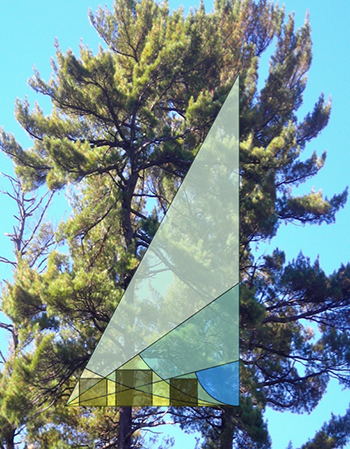This is a dynamic problem, and each slide catches it in three positions. The problem is elegant in its economy, consisting of a right triangle, a circular arc, and a square, one each of the three most fundamental shapes of geometry. It was painted as the only problem on a small sangaku that was saved from a temple that was destroyed in 1864. It wound up in the ceiling of a room of the municipal building in the city of Susaka, where it was discovered in 1997. I call it the Susaka Triangle.

While the first slide was sharp and clear, reaching for the sky, the second is muted and a bit murky, with some details only emerging after careful inspection. The first slide presented the problem, but the one below also presents the solution, which will be explained below.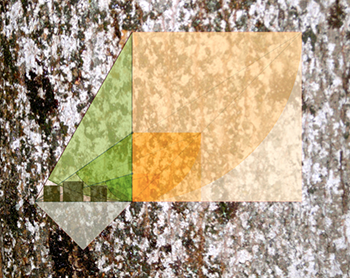You may want to go back to the first slide, and then compare to see what has been added here.

Can you see what the problem might ask? See below when you’re ready to explore it.

C. The Problem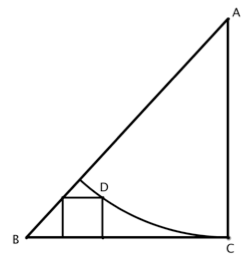The problem involves a right triangle ABC, with a circular arc drawn through C with center A, and a square on the base of the triangle with one corner on the arc and one on the hypotenuse. The main problem involves maximizing the square by varying the height of the triangle. But first let’s consider the construction. It is easy to construct the right triangle and the arc, but how do you precisely locate the point D, the upper right corner of the square? The second diagram shows how that is accomplished, by drawing the large square on the altitude of the triangle and connecting the vertex A to the far corner S of the large square. That line crosses the arc at D where the corner of the small square is located.

The reason this works is that the whole figure is similar to (has the same shape as) the small red trapezoid.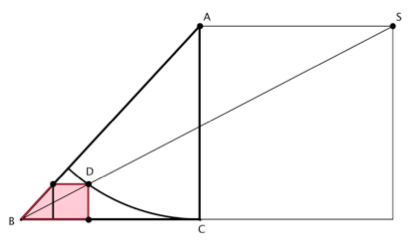Now suppose that we keep the base of the triangle fixed and vary the altitude. When the triangle is short, the square is squeezed beneath the hypotenuse, and when the triangle is tall the square is squeezed beneath the arc. Somewhere in between the square is maximized. When does that happen, and how big is the square. That is the central problem. Did you guess it from looking at the slides? A dynamic version, in which you can vary the height of the triangle and watch how the blue square responds, can be found at www.geogebratube.org/student/m8491.

C. The Solution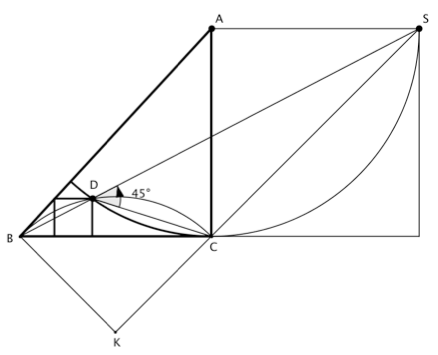If you increase the height of the triangle and trace the path of the point D , it will appear to travel in a circular arc from C to B. We will now show that D always lies on a circular arc whose center is at K, where BCK is a 45 degrees right triangle. The Inscribed Angle Theorem shows that ∠CDS=45 degrees, because ∠CAS=90 degrees. Hence ∠BDC=135 degrees, and then the converse of the Inscribed Angle Theorem shows that the point D lies on the circular arc that goes from B to C, with center at K. This means that the square will be largest when D is at the top of this arc, halfway between B and C. The next diagram illustrates this case.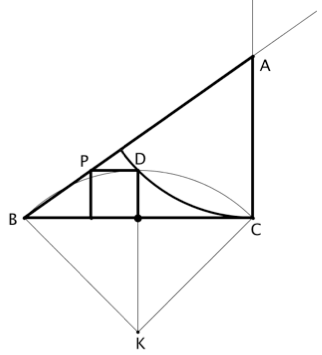The above figures were constructed in the order triangle-arc-square. This diagram was drawn in the reverse order, starting with the base BC, and then locating the point D at the midpoint of the arc BC, whose center is K. This allows us to draw the square on the base with upper right corner at D, and we call the upper left corner P. Then the peak of the triangle, the vertex A, is found where a ray from B through P crosses the vertical line through C. The diagram is now easily completed by drawing the triangle and the arc, and we are happy and relieved to see that the arc does indeed pass through the point D.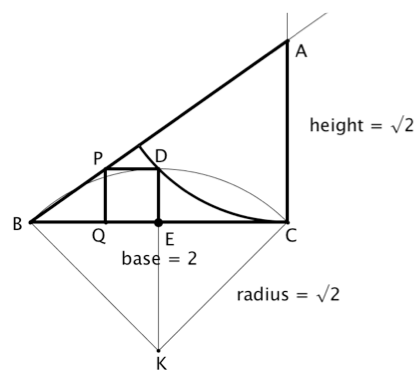From this diagram we can find size of the square and the height of the triangle. It will simplify computations a bit if we set the base of the triangle to 2. Then the radius KC equals √2, and since KE = 1, the height of the square is DE = KD - KE = √2−1≅0.414.
This means that for any base of the triangle, the ratio of the side of the maximal triangle to the base is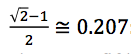the largest square is about one fifth of the base.

To find the height of the triangle, we can use the fact that out triangle ABC is similar to PBQ. Since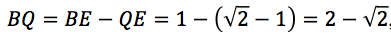and BC = 2.

We have: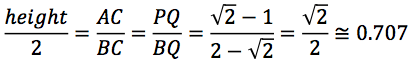So when the base is 2, the height is ￼√2, and for any fixed base, when the square is maximized the height of the triangle is about 70% of the base. Notice that this means that the square is maximized when the two arcs, one centered at A and the other at K, have the same radius.

This geometric solution was not found by the Temple Geometers, who preferred to compute. Instead, they derived an expression for the size of the square in terms of the height of the triangle and maximized the square using their limited version of differential calculus. The solution given here, credited by Fukagawa & Rothman to John F. Rigby of Cardiff University in Wales, is a gem of geometric thinking, a good note on which to close.

Epilogue: Freedom of Choice
JTG shows us that we could have a choice about how to approach mathematics: rather than looking for usefulness, we might be seeking beauty and meaning. Our schools rarely give us that choice, and most teachers of math are unaware of it.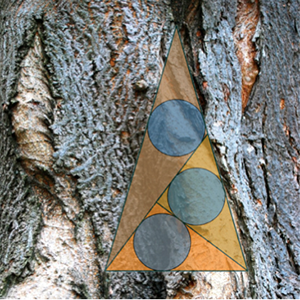Beyond that, choices abound that are rarely mentioned in polite mathematical company. You can’t choose to make 2 + 2 = 5 or π = 3, because arithmetic and geometry arise from counting and measuring, which are structured by time and space. But beyond that mathematics is done by choosing definitions and deriving necessary consequences of those definition. Mathematicians tend to remember the necessity and forget the choices. There are serious choices to be made at the most basic levels. What is an integer? What is a set? What is logic?

I am currently working on a book The Mathematics Revolution That Failed, which describes how mathematics might look if the program that Errett Bishop introduced at the 1966 International Congress of Mathematicians had gained traction. It would be a world where choices abound and are emphasized rather than hidden. Unfortunately, those drawn to mathematics often are attached to certainty, to absolute TRUTH, and are uncomfortable with choices. Perhaps mathematics is too important to be left to the mathematicians.

<< Art & Spirit in Mathematics: The Lessons of Japanese Temple Geometry (part II)